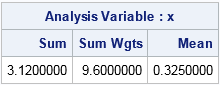Weighted averages are all around us. Teachers use weighted averages to assign a test more weight than a quiz. Schools use weighted averages to compute grade-point averages. Financial companies compute the return on a portfolio as a weighted average of the component assets. Financial charts show (linearly) weighted moving averages or exponentially-weighted moving averages for stock prices.

The weighted average (or weighted mean, as statisticians like to call it) is easy to compute in SAS by using either PROC MEANS or PROC UNIVARIATE. Use the WEIGHT statement to specify a weight variable (w), and use the VAR statement as usual to specify the measurement variable (x). The formula for the weighted mean is the ratio of sums Σ wixi /  Σ wi. The following example computes the numerator (weighted sum), the denominator (sum of weights), and the weighted mean for a set of eight data points. For these data and weights, the weighted sum is 0.325:

```data Wt; input x wt; datalines; -2 1 -1.5 0.8 -1.2 0.5 -0.5 1 0 1 0.8 1.5 1.4 2.3 2.0 1.5 ;   proc means data=Wt sum sumwgt mean; weight wt; var x; run;```The WEIGHT statement is supported in many SAS procedures. By convention, weights are positive values, so any observations that contain missing or nonpositive weights are excluded from the computation.

### Weighted means in SAS/IML software

The computation of the weighted mean is easy to program in SAS/IML software. Recall that the elementwise multiplication operator (#) computes the elementwise product of two vectors. If there are no missing values in the data and all the weights are positive, then the SAS/IML statement m = WtMean = sum(x#w) / sum(w) computes the weighted mean of the X values weighted by W.

For consistency with the rest of SAS, the following function excludes observations for which the X value is missing or for which the weight variable is not positive. Consequently, the following function duplicates the computation is used by PROC MEANS and PROC UNIVARIATE:

```proc iml; start WtMean(x, w); idx = loc(x^=. & w>0); /* use only valid observations */ if ncol(idx)=0 then return(.); /* no valid obs; return missing */ m = sum(x[idx]#w[idx]) / sum(w[idx]); /* compute weighted mean */ return( m ); finish;   use Wt; read all var {x wt}; close Wt; /* read the example data */ WtMean = WtMean(x, wt); /* test the function */ print WtMean;   call symputx("xbar", WtMean); /* store value in macro var for later */ quit;```

The result (not shown) is the same as reported by PROC MEANS. The SYMPUTX call creates a macro variable xbar that contains the value of the weighted mean for this example. This macro variable is used in the next section.

### Visualizing a weighted mean

Weighted distributions are not always easy to visualize, and for this reason PROC UNIVARIATE does not support creating graphs of weighted analyses. However, weighted means have a simple physical interpretation.

For the usual unweighted mean, imagine placing N identical point masses at the locations x1, x2, ..., xN along a massless rod. (An idealized point mass has no extent; the mass is concentrated at a single mathematical point.) The mean value of the X values is the center of mass for the point masses: the location at which the rod is perfectly balanced. In a similar way, the weighted mean is the location of the center of mass for a system of N point masses in which the mass wi is placed at the locations xi.

You can use a bubble plot to depict the physical arrangement of masses for this example. Instead of an idealized point mass, the bubble plot enables you to represent each mass by a circle whose size is related to the mass. The SIZE= option for the BUBBLE statement in PROC SGPLOT determines the diameter of the bubbles, but mass is proportional to area (actually volume, but I'm going to use a 2-D picture), so I use the square root of the weight to determine the size of each bubble. This trick ensures that the area of the bubbles is proportional to the weight.

The following DATA step computes the square root of each weight and adds a horizontal coordinate, y=0. The call to PROC SGPLOT creates the bubble plot. The REFLINE statement displays the massless rod. A drop line is shown at the center of mass for this system; the horizontal position is the valueof the xbar macro variable that was previously computed. (You can imagine that the system is perfectly balanced on the tip of a needle.) Finally, the TEXT statement (added in SAS 9.4m2) displays the weight of each mass. For earlier releases of SAS, you can use the MARKERCHAR= option in the SCATTER statement to display the weights.

```data Bubble; set Wt; y = 0; radius = sqrt(Wt); run;   ods graphics / width = 400px height=200px; proc sgplot data=Bubble noautolegend; refline 0 / axis=y; dropline x=&xbar y=0 / dropto=x; bubble x=x y=y size=radius; text x=x y=y text=wt / strip; /* or scatter x=x y=y / markerchar=wt; */ yaxis display=none; run;```In the graph, the five small masses to the left of the center of mass are balanced by the three larger masses to the right of the center of mass.

Although this example is one-dimensional, you can use the weighted mean computation to compute the center of mass for a two-dimensional collection of point masses: the X coordinates of the points are used to compute the X coordinate of the center of mass, and he Y coordinate for the center of mass is computed similarly. The bubble plot is easily modified to represent the two-dimensional arrangement.

In summary, the weighted mean is easy to compute and fun to visualize in SAS. Have you needed to compute a weighted mean? What did the weights represent? Leave a comment.

The post Compute a weighted mean in SAS appeared first on The DO Loop.